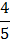# In the adjoining figure the equivalent resistance between A and B isa) 5b) 8c) 2.5d) 6.8## Question ID - 150264 :- In the adjoining figure the equivalent resistance between A and B isa) 5b) 8c) 2.5d) 6.83537

(a)

Equivalent circuit of the given circuit isBetween points C and D resistors 2, 2and 2are in series, therefore, their equivalent resistance,Resistors R’ and 6are in parallel, therefore their equivalent resistance is given byNow between points A and B 1, 3and 1are in series.

Therefore, resultant resistance is

R=1+3+1=5Next Question :
 If the ratio of the concentration of electron to that of holes in a semiconductor isand the ratio of current is, then what is the ratio of their drift velocities a)b)c)d)